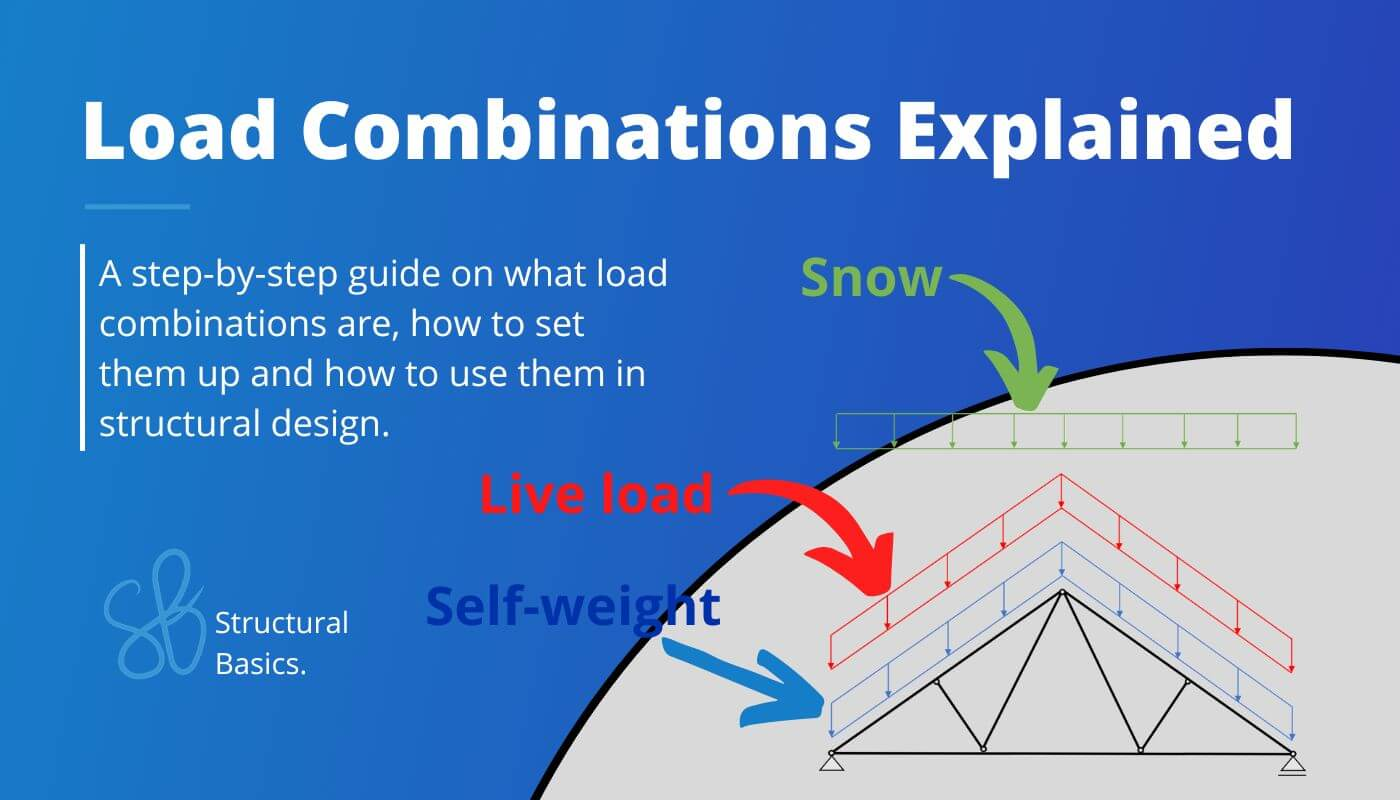# What are Load Combinations and how to calculate them?Last updated: June 6th, 2023

As buildings and structures must withstand the heaviest storms, accidental events and combined loading scenarios, engineers multiply loads with safety factors and combine different loads in so-called Load combinations to make sure that the structure doesn’t collapse.

We’ll show step-by-step, how load combinations work, what different types we use and how to calculate them.

Before we start: Don’t forget to check out our load combination generator! 🚀🚀

Load combinations combine different loads like snow, wind, dead, seismic and live load to represent a “real scenario”. A real scenario is for example the resulting force for a heavy wind storm. By setting up all possible load combinations we will find the worst-case scenario for a structural member which is in many cases the biggest load.

Load combinations according to Eurocode consist mostly of 3 components:

• Partial factor $\gamma$
• Factor for combination value of variable loads $\Psi_{0}$

So let’s look at an example 😎

## Loads acting on a flat roof – Example

Example flat roof

First, let’s define some symbols and values for our loads.

ULS stands for ultimate limit state. Due to ULS load combinations, structural members are designed for bending, shear, buckling, etc.

If you want to save time, then check out our load combination generator which automatically creates the ULS load combinations. 🔥🔥

According to Eurocode EN 1990 (6.10) the load combinations can be written as

Where

For the case of the flat roof, we get the following values

Which we now can put in the Load combinations.

If you are unsure of how to apply the different loads to roofs, then check out my post about loads and how to apply them (link)😁

Puhhh that was quite a lot of typing. Forgive me if I did a typo somewhere 🙏

Don’t worry, you do not have to do this manually every time because luckily most FE programs do that for us.

But if we were to dimension a timber beam for bending now manually we would use the biggest value of the Load combinations which is 4.0 kN/m2 (LC3) and transform it first in a line load (kN/m)

Assuming that the beams have a spacing of 0.8m we get the following line load:

$$4.0 \frac{kN}{m^2} * 0.8 m = 3.2 \frac{kN}{m}$$

That line load can now be applied to our static system

Perfect. So now we can go ahead and dimension a beam in the next blog post.

SLS stands for Serviceability limit state. Due to SLS load combinations structural members are designed for deflection, cracks in concrete, …

First, let’s look at the characteristic SLS load combination.

According to EN 1990 (6.14b) the characteristic load combinations can be written as

.. and if we put in the values, we get ..

Now let’s move on to the quasi-permanent SLS load combination.

According to EN 1990 (6.16b) the quasi-permanent load combinations can be written as.

Where

.. and if we put in the values, we will get ..

Alright, this post got longer than I thought 😅

We haven’t covered the accidental and frequent load combinations, but once we have an example where we need them we will also explain them.

With the knowledge we have got now, we can dimension our first timber beam in the next post. Hope to see you there 😁